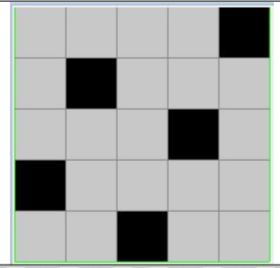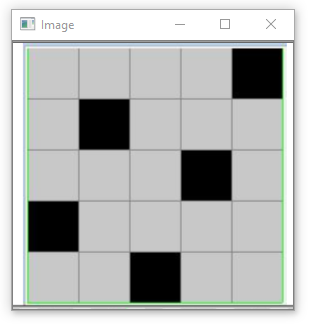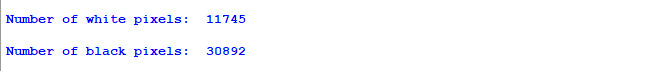# OpenCV – Counting the number of black and white pixels in the image

• Last Updated : 22 Jan, 2021

In this article, we will discuss counting the number of black pixels and white pixels in the image using OpenCV and NumPy.

Prerequisites:

The image we are using for the demonstration is shown below:To display OpenCV provides the imshow() method for displaying the image we have recently read.

#### Counting Pixels

NumPy provides a function sum() that returns the sum of all array elements in the NumPy array. This sum() function can be used to count the number of pixels on the basis of the required criteria.

Now, a bit of knowledge of the pixel patterns comes into the picture. As we know that each pixel in a coloured image ranges from [0-255] all-inclusive, the pixel value for the black color being 0 and that for the white color is 255. This gives us a certain fixed condition of differentiation for the black and white pixels respectively from the other color pixels respectively.

This condition can be written in the NumPy as:

number_of_white_pix = np.sum(img == 255)      # extracting only white pixels

number_of_black_pix = np.sum(img == 0)          # extracting only black pixels

The first line says to extract and count all pixels from cv2 image object “img” whose pixel value is 255 i.e. white pixels. Similarly, the second line says to extract and count all pixels from cv2 image object “img” whose pixel value is 0 i.e. black pixels.

The condition inside sum() extracts only those pixels from the image which follow that condition and assigns them value True (1) and rest of the pixels are assigned False (0). Thus, the sum of all True (1s) gives us the count of those pixels that are satisfying the given condition within the parenthesis.

Code: Python implementation to count the number of black and white pixels in the image using OpenCV

## Python3

 `# importing libraries ` `import` `cv2 ` `import` `numpy as np ` ` `  `# reading the image data from desired directory ` `img ``=` `cv2.imread(``"chess5.png"``) ` `cv2.imshow(``'Image'``,img) ` ` `  `# counting the number of pixels ` `number_of_white_pix ``=` `np.``sum``(img ``=``=` `255``) ` `number_of_black_pix ``=` `np.``sum``(img ``=``=` `0``) ` ` `  `print``(``'Number of white pixels:'``, number_of_white_pix) ` `print``(``'Number of black pixels:'``, number_of_black_pix)`

Output:Image to ProcessCount of black and white pixels from the above image

Thus, we can infer from the above results that the image can be processed to retrieve the pixels of any desired color with the help of their color code or obtain the pixels based on any other condition as required.

#### References:

My Personal Notes arrow_drop_up
Recommended Articles
Page :